Suppose you built a scale-model atom in which the nucleus is the size of a tennis ball. About how far would the cloud of electrons extend?

Question

Suppose you built a scale-model atom in which the nucleus is the size of a tennis ball. About how far would the cloud of electrons extend?

in progress 0
5 months 2021-08-11T10:58:17+00:00 1 Answers 3 views 0

500 m

Explanation:

The size of a nucleus is about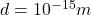Instead, the size of the electron cloud extends at the order of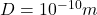This means that the ratio between the size of the electron cloud and the size of the nucleus is about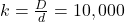So, the electron cloud is about 10,000 bigger than the nucleus of the atom.

Here we want to build a scale-model atom, in which the nucleus is the size of a tennis ball, so a size of approximately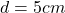Therefore, since the proportions must be respected, the electron clouds must be 10,000 bigger, so its size in this model would be: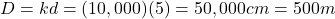So, the size of the electron cloud would be 500 m.